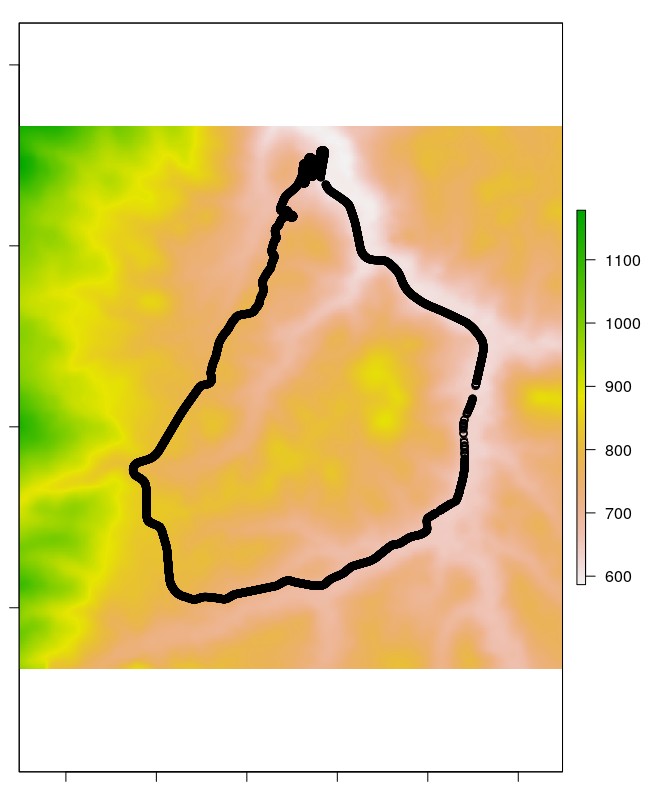## Introduction

This article aims to provide external verification of the results provided by the package. So far only one verification dataset has been used, but we hope to find others. If you know of verification datasets, please let us know — initially we planned to use a dataset from a paper on river slopes (Cohen et al. 2018), but we could find no way of extracting the underlying data to do the calculation.

For this article we primarily used the following packages, although others are loaded in subsequent code chunks.

``````library(slopes)
library(sf)
#> Linking to GEOS 3.10.2, GDAL 3.4.1, PROJ 8.2.1; sf_use_s2() is TRUE``````

The results are reproducible (requires downloading input data manually and installing additional packages). To keep package build times low, only the results are presented below.

## Three-dimensional traces of roads dataset

An input dataset, comprising a 3D linestring recorded using a dual frequency GNSS receiver (a Leica 1200) with a vertical accuracy of 20 mm (Ariza-López et al. 2019) was downloaded from the figshare website as a .zip file and unzipped and inflated in the working directory as follows (not evaluated to reduce package build times):

``````download.file("https://ndownloader.figshare.com/files/14331185", "3DGRT_AXIS_EPSG25830_v2.zip")
unzip("3DGRT_AXIS_EPSG25830_v2.zip")
plot(trace)
nrow(trace)
#> 11304
summary(trace\$X3DGRT_h)
#>  Min. 1st Qu.  Median    Mean 3rd Qu.    Max.
#>   642.9   690.3   751.4   759.9   834.3   884.9 ``````

To verify our estimates of hilliness, we generated slope estimates for each segment and compared them with Table 7 in Ariza-López et al. (2019). The absolute gradient measure published in that paper were:

``````res_gps = c(0.00, 4.58, 1136.36, 6.97)
res_final = c(0.00, 4.96, 40.70, 3.41)
res = data.frame(cbind(
rbind(res_gps, res_final)
))
names(res) = c("Source", "min", " mean", " max", " stdev")
knitr::kable(res, row.names = FALSE)``````
Source min mean max stdev
GPS 0 4.58 1136.36 6.97
Dual frequency GNSS receiver 0 4.96 40.7 3.41
``````# mapview::mapview(trace) # check extent: it's above 6km in height
# remotes::install_github("hypertidy/ceramic")
loc = colMeans(sf::st_coordinates(sf::st_transform(trace, 4326)))
e = ceramic::cc_elevation(loc = loc[1:2], buffer = 3000)
trace_projected = sf::st_transform(trace, 3857)
plot(e)The slopes were estimated as follows:

``````# source: https://www.robinlovelace.net/presentations/munster.html#31
points2line_trajectory = function(p) {
c = st_coordinates(p)
i = seq(nrow(p) - 2)
l = purrr::map(i, ~ sf::st_linestring(c[.x:(.x + 1), ]))
lfc = sf::st_sfc(l)
a = seq(length(lfc)) + 1 # sequence to subset
p_data = cbind(sf::st_set_geometry(p[a, ], NULL))
sf::st_sf(p_data, geometry = lfc)
}
r = points2line_trajectory(trace_projected)
# summary(st_length(r)) # mean distance is 1m! Doesn't make sense, need to create segments
s = slope_raster(r, e = e)
slope_summary = data.frame(min = min(s), mean = mean(s), max = max(s), stdev = sd(s))
slope_summary = slope_summary * 100
knitr::kable(slope_summary, digits = 1)``````
min mean max stdev
0 6.2 48.2 5.6

Combined with the previous table from Ariza-López et al. (2019), these results can be compared with those obtained from mainstream GPS, and an accurate GNSS receiver:

Source min mean max stdev
GPS 0 4.58 1136.36 6.97
Dual frequency GNSS receiver 0 4.96 40.7 3.41
Slopes R package 0 6.2 48.2 5.6

It is notable that the package substantially overestimates the gradient, perhaps due to the low resolution of the underlying elevation raster. However, the slopes package seems to provide less noisy slope estimates than the GPS approach, with lower maximum values and low standard deviation.

Ariza-López, Francisco Javier, Antonio Tomás Mozas-Calvache, Manuel Antonio Ureña-Cámara, and Paula Gil de la Vega. 2019. “Dataset of Three-Dimensional Traces of Roads.” Scientific Data 6 (1): 1–10. https://doi.org/10.1038/s41597-019-0147-x.
Cohen, Sagy, Tong Wan, Md Tazmul Islam, and J. P. M. Syvitski. 2018. “Global River Slope: A New Geospatial Dataset and Global-Scale Analysis.” Journal of Hydrology 563 (August): 1057–67. https://doi.org/10.1016/j.jhydrol.2018.06.066.